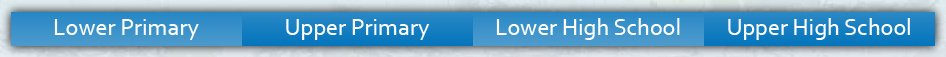Lower High School Enter keywords to search by topic or worksheet description

Worksheets in this category provide students with practice in re-arranging and solving linear equations.

Worksheet Name Files
Solving One-Step Equations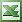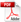One Step Equations Involving Decimals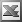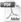Solving Two or Three Step EquationsSolving Equations - Algebraic FractionsSolving Equations with Variables on Both SidesSimultaneous Equations (Slope-Intercept Form)Simultaneous Equations (Both Forms)Literal EquationsNumber Patterns: Algebraic Relationships